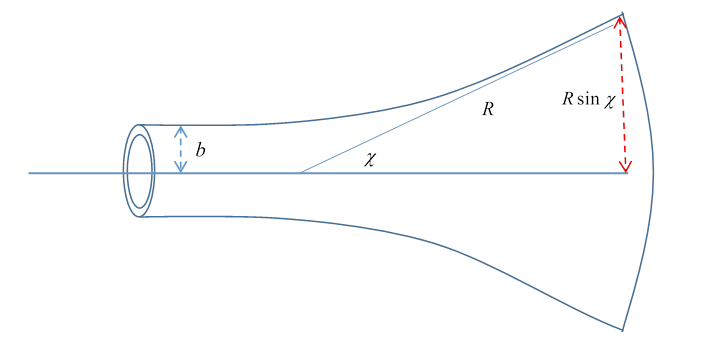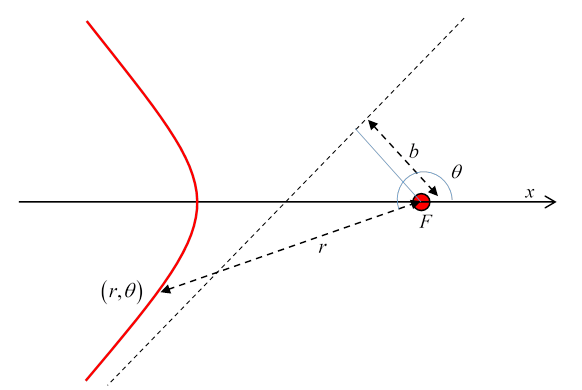# 16. Elastic Scattering

Michael Fowler

## Billiard Balls

“Elastic” means no internal energy modes of the scatterer or of the scatteree are excited$—$so total kinetic energy is conserved.  As a simple first exercise, think of two billiard balls colliding. The best way to see it is in the center of mass frame of reference.  If they’re equal mass, they come in from opposite directions, scatter, then move off in opposite directions.  In the early days of particle accelerators (before colliders) a beam of particles was directed at a stationary target.  So, the frame in which one particle is initially at rest is called the lab frame.  What happens if we shoot one billiard ball at another which is initially at rest?  (We’ll ignore possible internal energies, including spinning.)  The answer is that they come off at right angles. This follows trivially from conservation of energy and momentum (in an obvious notation)

$m\stackrel{\to }{v}=m{\stackrel{\to }{v}}_{1}+m{\stackrel{\to }{v}}_{2},\text{ }\frac{1}{2}m{\stackrel{\to }{v}}_{}^{2}=\frac{1}{2}m{\stackrel{\to }{v}}_{1}^{2}+\frac{1}{2}m{\stackrel{\to }{v}}_{2}^{2}$

and Pythagoras’ theorem.

## Discovery of the Nucleus

The first significant use of scattering to learn about the internal structure of matter was Rutherford’s use of $\alpha$ particles directed at gold atoms.  This experiment revealed the atomic nucleus for the first time. Our plan here is to analyze this kind of scattering, to understand why it indicated the presence of a nucleus.  Similar much later analyses have established that the proton itself has point like constituents, so this is not just of distant historical interest.

For $\alpha$ particles on gold atoms, it’s an excellent approximation to take the scatterer as being fixed.  This is not an essential requirement, but it simplifies the calculation, and can be corrected for later.

To visualize what’s going on, think of the scatterer as a bowling ball with tiny marbles directed towards it, they’re moving fast horizontally, along parallel but random paths.  (Let’s take zero gravity here$—$the $\alpha$ particles we’re modeling are moving at about one-twentieth the speed of light!)

We observe the rate at which marbles are being scattered in various directions.  Call the scattering angle $\chi .$

So, let’s assume the width of the “beam” of marbles is much greater than the size of the bowling ball. We’ll also take the intensity of the beam to be uniform, with $n$ marbles crossing unit area perpendicular to the beam per second.

Now, if the bowling ball has radius $R,$ and we ignore the radius of the tiny marbles, the number of marbles that strike the bowling ball and are scattered is clearly $\pi {R}^{2}n$ per second.  Not surprisingly, $\pi {R}^{2}$ is called the total cross-section and usually denoted by $\sigma .$

## The Differential Cross Section

In a real scattering experiment, information about the scatterer can be figured out from the different rates of scattering to different angles.  Detectors are placed at various angles $\left(\theta ,\varphi \right)$.  Of course, a physical detector collects scattered particles over some nonzero solid angle.  The usual notation for infinitesimal solid angle is $d\Omega =\mathrm{sin}\theta d\theta d\varphi .$ The full solid angle (all possible scatterings) is $\int d\Omega =4\pi$, the area of a sphere of unit radius. (Note: Landau uses $dο$ for solid angle increment, but $d\Omega$ has become standard.)

The differential cross section, written $d\sigma /d\Omega ,$ is the fraction of the total number of scattered particles that come out in the solid angle $d\Omega .$, so the rate of particle scattering to this detector is $nd\sigma /d\Omega$, with $n$ the beam intensity as defined above.

Now, we’ll assume the potential is spherically symmetric.  Imagine a line parallel to the incoming particles going through the center of the atom.  For a given ingoing particle, its impact parameter is defined as the distance its ingoing line of flight is from this central line. Landau calls this $\rho ,$ we’ll follow modern usage and call it $b.$

A particle coming in with impact parameter between $b$ and $b+db$ will be scattered through an angle between $\chi$ and $\chi +d\chi ,$ where we’re going to calculate $\chi \left(b\right)$ by solving the equation of motion of a single particle in a repulsive inverse-square force.

Note: we’ve switched for this occasion from $\theta$ to $\chi$ for the angle scattered through because we want to save $\theta$ for the $\left(r,\theta \right)$ coordinates describing the complete trajectory, or orbit, of the scattered particle.So, an ingoing cross section $d\sigma =2\pi bdb$ scatters particles into an outgoing spherical area (centered on the scatterer) $2\pi R\mathrm{sin}\chi Rd\chi ,$ that is, a solid angle $d\Omega =2\pi \mathrm{sin}\chi d\chi .$

Therefore the scattering differential cross section

$\frac{d\sigma }{d\Omega }=\frac{b\left(\chi \right)}{\mathrm{sin}\chi }\left|\frac{db}{d\chi }\right|.$

(Note that $d\chi /db$ is clearly negative$—$increasing $b$ means increasing distance from the scatterer, so a smaller $\chi$.)

## Analyzing Inverse-Square Repulsive Scattering:  Kepler Again

To make further progress, we must calculate $b\left(\chi \right)$, or equivalently $\chi \left(b\right):$ what is the angle of scattering, the angle between the outgoing velocity and the ingoing velocity, for a given impact parameter? $\chi$ will of course also depend on the strength of the repulsion, and the ingoing particle energy.

Recall our equation for Kepler orbits:

$\frac{{d}^{2}u}{d{\theta }^{2}}+u=\frac{GM{m}^{2}}{{L}^{2}}.$

Let’s now switch from gravitational scattering with an attractive force $GMm/{r}^{2}$ to an electrical repulsive force between two charges ${Z}_{1}e,\text{ }{Z}_{2}e$, force strength $\frac{1}{4\pi {\epsilon }_{0}}\frac{{Z}_{1}{Z}_{2}{e}^{2}}{{r}^{2}}=\frac{k}{{r}^{2}},$ say.  Since this is repulsive, the sign will change in the radial acceleration equation,

$\frac{{d}^{2}u}{d{\theta }^{2}}+u=-\frac{km}{{L}^{2}}.$

Also, we want the scattering parameterized in terms of the impact parameter $b$ and the incoming speed ${v}_{\infty },$ so putting $L=m{v}_{\infty }b$ this is

$\frac{{d}^{2}u}{d{\theta }^{2}}+u=-\frac{k}{m{b}^{2}{v}_{\infty }^{2}}.$

So just as with the Kepler problem, the orbit is given by

$\frac{1}{r}=u=-\frac{k}{m{b}^{2}{v}_{\infty }^{2}}+C\mathrm{cos}\left(\theta -{\theta }_{0}\right)=-\kappa +C\mathrm{cos}\left(\theta -{\theta }_{0}\right),$ say.

From the lecture on Orbital Mathematics, the polar equation for the left hyperbola branch relative to the external (right) focus is

$\mathcal{l}/r=-e\mathrm{cos}\theta -1,$

this is a branch symmetric about the $x$ axis:But we want the incoming branch to be parallel to the axis, which we do by suitable choice of ${\theta }_{0}$.

In other words, we rotate the hyperbola clockwise through half the angle between its asymptotes, keeping the scattering center (right-hand focus) fixed.

From the lecture on orbital mathematics (last page), the perpendicular distance from the focus to the asymptote is the hyperbola parameter $b$!   Presumably, this is why we use $b$ for the impact parameter.

Hence the particle goes in a hyperbolic path with parameters $e/\mathcal{l}=-C,\text{ }1/\mathcal{l}=\kappa .$ This is not enough information to fix the path uniquely: we’ve only fed in the angular momentum $mb{v}_{\infty },$ not the energy, so this is a family of paths having different impact parameters but the same angular momentum .

We can, however, fix the path uniquely by equating the leading order correction to the incoming zeroth order straight path: the particle is coming in parallel to the $x$ axis far away to the left, perpendicular distance $b$ from the axis, that is, from the line $\theta =\pi .$  So, going back to that pre-scattering time,

$u\to 0,\text{ }\text{ }\pi -\theta \to b/r=bu,$and in this small $u$ limit,

$u=C\mathrm{cos}\left(\pi -bu-{\theta }_{0}\right)-\kappa \cong C\mathrm{cos}\left(\pi -{\theta }_{0}\right)-\kappa +bCu\mathrm{sin}\left(\pi -{\theta }_{0}\right).$

Matching the zeroth order and the first order terms

$C\mathrm{cos}\left(\pi -{\theta }_{0}\right)=\kappa ,\text{ }u=bCu\mathrm{sin}\left(\pi -{\theta }_{0}\right),$

eliminates $C$ and fixes the angle ${\theta }_{0},$ which is the angle the hyperbola had to be rotated through to align the asymptote with the negative $x$ axis, and therefore half the angle between the asymptotes, which would be $\pi$ minus the angle of scattering $\chi$ (see the earlier diagram),

$\mathrm{tan}\left(\pi -{\theta }_{0}\right)=-\mathrm{tan}{\theta }_{0}=\frac{1}{b\kappa }=\frac{mb{v}_{\infty }^{2}}{k}.$

$\chi =\pi -2{\mathrm{cot}}^{-1}b\kappa =2{\mathrm{tan}}^{-1}b\kappa ,$

So this is the scattering angle in terms of the impact parameter $b$, that is, in the diagram above

$\chi \left(b\right)=2{\mathrm{tan}}^{-1}\left(\frac{k}{mb{v}_{\infty }^{2}}\right).$

Equivalently,

$b=\frac{k}{m{v}_{\infty }^{2}}\mathrm{cot}\frac{\chi }{2}$, so $db=\frac{k}{2m{v}_{\infty }^{2}}{\text{cosec}}^{2}\frac{\chi }{2}d\chi ,$

and the incremental cross sectional area

$d\sigma =2\pi bdb=\pi {\left(\frac{k}{m{v}_{\infty }^{2}}\right)}^{2}{\text{cosec}}^{2}\frac{1}{2}\chi \mathrm{cot}\frac{1}{2}\chi d\chi =\pi {\left(\frac{k}{m{v}_{\infty }^{2}}\right)}^{2}\frac{\mathrm{cos}\frac{1}{2}\chi }{{\mathrm{sin}}^{3}\frac{1}{2}\chi }d\chi ={\left(\frac{k}{2m{v}_{\infty }^{2}}\right)}^{2}\frac{1}{{\mathrm{sin}}^{4}\frac{1}{2}\chi }d\Omega .$ .

This is Rutherford’s formula: the incremental cross section for scattering into an incremental solid angle, the differential cross section

$\frac{d\sigma }{d\Omega }={\left(\frac{k}{2m{v}_{\infty }^{2}}\right)}^{2}\frac{1}{{\mathrm{sin}}^{4}\frac{1}{2}\chi }.$

(Recall $k=\frac{1}{4\pi {\epsilon }_{0}}{Z}_{1}{Z}_{2}{e}^{2}$ in MKS units.)

## Vectorial Derivation of the Scattering Angle

(from Milne)

The essential result of the above analysis was the scattering angle as a function of impact parameter, for a given incoming energy.  It's worth noting that this can be found more directly by vectorial methods from Hamilton's equation.

Recall from the last lecture Hamilton’s equation

$\stackrel{\to }{L}×m\stackrel{¨}{\stackrel{\to }{r}}=-m{r}^{2}f\left(r\right)\frac{d\stackrel{^}{\stackrel{\to }{r}}}{dt}$

and the integral for an inverse square force $f\left(r\right)=k/{r}^{2}$ (changing the sign of $\stackrel{\to }{A}$ for later convenience)

$\stackrel{\to }{L}×m\stackrel{˙}{\stackrel{\to }{r}}=km\text{ }\stackrel{^}{\stackrel{\to }{r}}+\stackrel{\to }{A}.$

As previously discussed, multiplying by $\stackrel{\to }{L}\cdot$ establishes that $\stackrel{\to }{A}$ is in the plane of the orbit, and multiplying by $\stackrel{\to }{r}\cdot$ gives

$-{L}^{2}=kmr+Ar\mathrm{cos}\theta$.

This corresponds to the equation

$\mathcal{l}/r=-e\mathrm{cos}\theta -1$

(the left-hand branch with the right-hand focus as origin, note from diagram above that $\mathrm{cos}\theta$ is negative throughout) and

$\frac{{L}^{2}}{kmr}=-1-\frac{A}{km}\mathrm{cos}\theta .$

To find the scattering angle, suppose the unit vector pointing parallel to the asymptote is ${\stackrel{^}{\stackrel{\to }{r}}}_{\infty },$ so the asymptotic velocity is ${v}_{\infty }{\stackrel{^}{\stackrel{\to }{r}}}_{\infty }.$

Note that as before, $\stackrel{\to }{A}$ is along the major axis (to give the correct form for the $\left(r,\theta \right)$ equation), and $r=\infty$ gives the asymptotic angles from

$\mathrm{cos}{\theta }_{r=\infty }=-km/A.$

We’re not rotating the hyperbola as we did in the alternative treatment above: here we keep it symmetric about the $x$ axis, and find its asymptotic angle to that axis, which is one-half the scattering angle.

Now take Hamilton’s equation in the asymptotic limit, where the velocity is parallel to the displacement:

the vector product of Hamilton’s equation $×\text{ }\text{\hspace{0.17em}}{\stackrel{^}{\stackrel{⇀}{r}}}_{\infty }$ yields

$\stackrel{\to }{A}×{\stackrel{^}{\stackrel{⇀}{r}}}_{\infty }=\left(\stackrel{\to }{L}×m{v}_{\infty }{\stackrel{^}{\stackrel{⇀}{r}}}_{\infty }\right)×{\stackrel{^}{\stackrel{⇀}{r}}}_{\infty }=-\stackrel{\to }{L}\left(L/b\right).$

It follows that

$\mathrm{sin}{\theta }_{r=\infty }=-{L}^{2}/Ab,$

And together with $\mathrm{cos}{\theta }_{r=\infty }=-km/A,$ we find

$\mathrm{tan}{\theta }_{r=\infty }=\frac{{L}^{2}}{kmb}=\frac{mb{v}_{\infty }^{2}}{k}.$

This is the angle between the asymptote and the major axis: the scattering angle

$\chi =\pi -2{\theta }_{r=\infty }=2\left(\frac{\pi }{2}-{\theta }_{r=\infty }\right)=2{\mathrm{tan}}^{-1}\left(\frac{k}{mb{v}_{\infty }^{2}}\right),$

agreeing with the previous result.